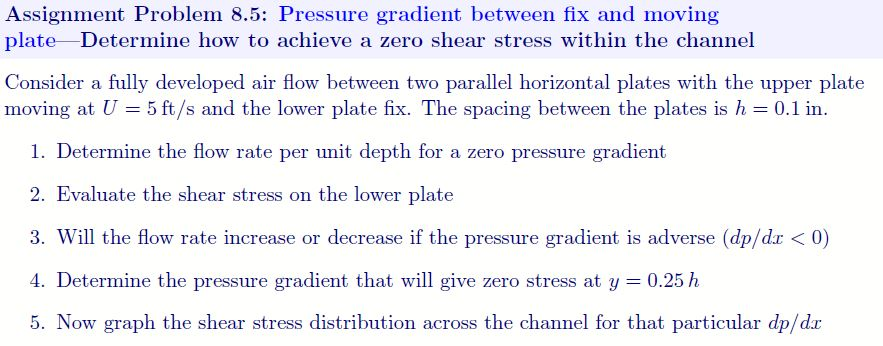# (Solved): Assignment Problem 8.5: Pressure Gradient Between Fix And Moving Plate Determine How To Achieve A Ze...Assignment Problem 8.5: Pressure gradient between fix and moving plate Determine how to achieve a zero shear stress within the channel Consider a fully developed air flow between two parallel horizontal plates with the upper plate moving at U = 5 ft/s and the lower plate fix. The spacing between the plates is h = 0.1 in. 1. Determine the flow rate per unit depth for a zero pressure gradient 2. Evaluate the shear stress on the lower plate 3. Will the flow rate increase or decrease if the pressure gradient is adverse (dp/dir <0) 4. Determine the pressure gradient that will give zero stress at y = 0.25 h 5. Now graph the shear stress distribution across the channel for that particular dp dr

We have an Answer from Expert